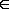Michelle D.

# A function y(t) satisfies the differential equation

A function y(t) satisfies the differential equation

dy/dx = y4 − 4y3 + 3y2

(a) What are the constant solutions of the equation? (Enter your answers as a comma-separated list.)

y= ??

(b) For what values of y is y increasing? (Enter your answer in interval notation.)

y=

(c) For what values of y is y decreasing? (Enter your answer in interval notation.)

y=